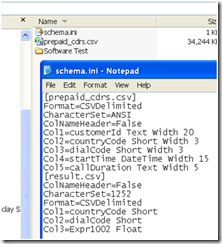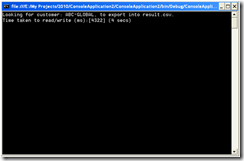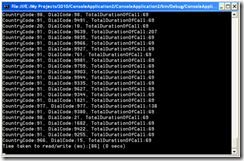## Feature Post

Sometime back a question was asked to develop a well performant parser– there was no restriction defined in the question whatsoever as to what technology, logic, flow/etc should be applied. Just the input block, and expected output result format, and maximum time that the parser may take.
• How to query a .CSV and save the result in another CSV?
• Custom .CSV Parser?
• How to ETL .CSV into a .CSV
• High performance .CSV parser
I found the performance requirement interesting, and so is the reason of this post; besides, another reason is to discuss only “some” of the solutions of the problem; therefore, it should be stated clearly in a question as to what exactly is required, just to save the the test’ee and tester from any after shocks. Though can have several solutions, but lets get into the details and see what do we have here. Following are important aspects of the problem.

Calculate the usage of different country and dial codes for a particular customer, and write result in a separate file.

Functional requirement:
• Read from CSV data source
• Query for the data (select, group by, sum, count, etc)
• Write into a separate .CSV file
Non functional requirement: (Performance)

Data source contains the ~1.2 million records, and the module is required to complete the whole procedure in less than 5 seconds.

Given that:

We have a CustomerData.CSV file already exists, to which we will query.

CustomerData.CSV has the following schema:

 Columns Description Field 1 This field contains the customer id (sorted in ascending order) Field 2 Country code Field 3 Dial Code Field 4 Start time Field 5 Call duration

Result.CSV has the following schema:

 Columns Description Field 1 Country code Field 2 Dial Code Field 3 Total duration (in minutes and seconds)

SOLUTION 1: (Use Jet OLE DB Text Driver)

Easiest, quickest, fastest, and very well performant!

Step 1: Define the following schema.ini file in some folder that you like:If you would like, then look into the Schema.ini File (Text File Driver). Following content goes in schema.ini:
`   1: [CustomerData.csv]`
`   2: Format=CSVDelimited`
`   3: CharacterSet=ANSI`
`   4: ColNameHeader=False`
`   5: Col1=customerId Text Width 20`
`   6: Col2=countryCode Short Width 3`
`   7: Col3=dialCode Short Width 3`
`   8: Col4=startTime DateTime Width 15`
`   9: Col5=callDuration Text Width 5`
`  10: [result.csv]`
`  11: ColNameHeader=False`
`  12: CharacterSet=1252`
`  13: Format=CSVDelimited`
`  14: Col1=countryCode Short`
`  15: Col2=dialCode Short`
`  16: Col3=Expr1002 Float`

STEP 2: Stub in the backend code in some .cs file
`   1: private static void Query(string CustomerID)`
`   2: {`
`   3:     //Pseudo/logic:`
`   4:     //Use jet ole db text driver to select * insert into new table; `
`   5:     //to read-from a .csv, and write-into a .csv`
`   6:  `
`   7:     string customerId = CustomerID;`
`   8:     string writeTo = @"result.csv";`
`   9:     string readFrom = @"CustomerData.csv";`
`  10:  `
`  11:     //1: SELECT * INTO NEW_TABLE `
`  12:     //2:  FROM SOURCE_TABLE `
`  13:  `
`  14:     //dont just read, write as well.`
`  15:     string query = @"  SELECT `
`  16:                     countryCode, dialCode, sum(callDuration) INTO " + writeTo + @"`
`  17:                 FROM `
`  18:                     [" + readFrom + @"] `
`  19:                 WHERE `
`  20:                     customerId='" + customerId + @"' `
`  21:                 GROUP BY countryCode, dialCode";`
`  22:  `
`  23:     Stopwatch timer = new Stopwatch();`
`  24:  `
`  25:     try`
`  26:     {`
`  27:         Console.WriteLine("Looking for customer: {0}, to export into {1}.", customerId, writeTo);`
`  28:                 `
`  29:         string connectionString = @"Provider=Microsoft.Jet.OLEDB.4.0;Data Source=E:\MyDocs\Software Test\;Extended Properties='text;HDR=No;FMT=CSVDelimited'";`
`  30:         using (OleDbConnection conn = new OleDbConnection(connectionString))`
`  31:         {`
`  32:             OleDbCommand cmd = new OleDbCommand(query, conn);`
`  33:             conn.Open();`
`  34:             timer.Start();`
`  35:             int nRecordsAffected = cmd.ExecuteNonQuery();`
`  36:             timer.Stop();`
`  37:             conn.Close();`
`  38:         }`
`  39:     }`
`  40:     catch (Exception ex) { Console.Write(ex.ToString()); }`
`  41:     Console.WriteLine("Time taken to read/write (ms):[{0}] ({1} secs)", timer.ElapsedMilliseconds, TimeSpan.FromMilliseconds(timer.ElapsedMilliseconds).Seconds);`
`  42: }`

Output:SOLUTION 2: (Write a custom class, create indexes, and apply bisection search)

For instance, following code performs following operations to achieve the same:
1. Perform indexing on the selected column
2. Select specific records from the .CSV file
3. Perform aggregate function, call SUM() – Use LINQ
Usage:
`   1: using (CsvParser parser = new CsvParser(@"E:\CustomerData.csv"))`
`   2: {`
`   3:     parser.PerformIndexing((int)CsvParser.CsvColumns.Col1_CustomerID);//One time only.`
`   4:  `
`   5:     timer.Start();`
`   6:     parser.Select("AMANTEL").Sum((int)CsvParser.CsvColumns.CallDuration);`
`   7:     timer.Stop();`
`   8:  `
`   9:     foreach (var o in parser.Result)`
`  10:     {`
`  11:         Console.WriteLine(string.Format("CountryCode:{0}, DialCode:{1}, TotalDurationOfCall:{2}",`
`  12:                    o.CountryCode, o.DialCode, o.TotalDurationOfCall));`
`  13:     }`
`  14:  `
`  15: }`
`  16: Console.WriteLine("Time taken to read/write (ms):[{0}] ({1} secs)", timer.ElapsedMilliseconds, TimeSpan.FromMilliseconds(timer.ElapsedMilliseconds).Seconds);`

Output:Backend code:
`   1: class CsvParser : IDisposable`
`   2: {`
`   3:  `
`   4:     public dynamic Result { get; set; }`
`   5:     private string _fileName;`
`   6:     private char _separator = ',';`
`   7:     private Dictionary<string, Bounds> _lstIndex = new Dictionary<string, Bounds>();`
`   8:     private List<string> _Rows = new List<string>();`
`   9:     public enum CsvColumns { Col1_CustomerID = 0, Col2_CountryCode = 1, Col3_DialCode = 2, Col4_StartTime = 3, CallDuration = 5 }`
`  10:  `
`  11:     //Simple bound structure to hold start and end index in the file.`
`  12:     class Bounds`
`  13:     {`
`  14:         public int Start { get; set; }`
`  15:         public int End { get; set; }`
`  16:  `
`  17:         public Bounds(int start, int stop) { Start = start; End = stop; }`
`  18:     }`
`  19:  `
`  20:  `
`  21:     //Default constructor`
`  22:     public CsvParser(string file, char seperator = ',')`
`  23:     {`
`  24:         if (string.IsNullOrEmpty(file)) throw new Exception("Invalid file");`
`  25:  `
`  26:         this._fileName = file; this._separator = seperator;`
`  27:     }`
`  28:  `
`  29:     /// <summary>`
`  30:     /// Should be called once, before using the object;`
`  31:     /// </summary>`
`  32:     /// <param name="nColumn">Column to be indexed</param>`
`  33:     /// <returns>Chained object</returns>`
`  34:     public CsvParser PerformIndexing(int nColumn)`
`  35:     {`
`  36:         using (StreamReader reader = new StreamReader(_fileName))`
`  37:         {`
`  38:             string previousVal = string.Empty; string currentVal = string.Empty;`
`  39:             int nStart = 0;`
`  40:             int nEnd = 0;`
`  41:             int nRowCounter = 0;`
`  42:  `
`  43:             do`
`  44:             {`
`  45:                 currentVal = reader.ReadLine().Split(_separator)[nColumn];`
`  46:  `
`  47:                 if (previousVal != currentVal)`
`  48:                 {`
`  49:                     if (!string.IsNullOrEmpty(previousVal))`
`  50:                     {`
`  51:                         nEnd = nRowCounter;`
`  52:                         _lstIndex.Add(previousVal, new Bounds(nStart, nEnd)); //Add previous value`
`  53:                         nStart = nEnd + 1;//next line`
`  54:                     }`
`  55:  `
`  56:                     previousVal = currentVal;`
`  57:                 }`
`  58:  `
`  59:  `
`  60:                 nRowCounter++;//next line`
`  61:             } while (!reader.EndOfStream);`
`  62:         }`
`  63:  `
`  64:         System.Diagnostics.Trace.WriteLine(string.Format("Done. Total indexed {0}.", _lstIndex.Count));`
`  65:         return this;`
`  66:     }`
`  67:  `
`  68:     /// <summary>`
`  69:     /// Select rows where given customer id`
`  70:     /// </summary>`
`  71:     /// <param name="CustomerID">Customer id predicate</param>`
`  72:     /// <returns></returns>`
`  73:     internal CsvParser Select(string CustomerID)`
`  74:     {`
`  75:         using (StreamReader reader = new StreamReader(_fileName))`
`  76:         {`
`  77:             //1. Get location from index; also get the next index id so that we know where to stop.`
`  78:             //2. Jump to that position`
`  79:             //3. Start fetching`
`  80:  `
`  81:             Bounds bounds = _lstIndex[CustomerID];`
`  82:  `
`  83:             int nRowCounter = 0;`
`  84:             while (!reader.EndOfStream || nRowCounter == bounds.End)`
`  85:             {`
`  86:                 if (nRowCounter >= bounds.Start)`
`  87:                     _Rows.Add(reader.ReadLine());`
`  88:  `
`  89:                 nRowCounter++;`
`  90:  `
`  91:                 if (nRowCounter > bounds.End) break;`
`  92:             }`
`  93:         }`
`  94:  `
`  95:         return this;`
`  96:     }`
`  97:  `
`  98:     /// <summary>`
`  99:     /// Binary search`
` 100:     /// </summary>`
` 101:     /// <param name="data"></param>`
` 102:     /// <param name="key"></param>`
` 103:     /// <param name="left"></param>`
` 104:     /// <param name="right"></param>`
` 105:     /// <returns></returns>`
` 106:     [Obsolete("Unused", true)]`
` 107:     internal static int Search(string[] data, string key, int left, int right)`
` 108:     {`
` 109:         if (left <= right)`
` 110:         {`
` 111:             int middle = (left + right) / 2;`
` 112:             if (key == data[middle])`
` 113:                 return middle;`
` 114:             else if (!key.Equals(data[middle]))`
` 115:                 return Search(data, key, left, middle - 1);`
` 116:             else`
` 117:                 return Search(data, key, middle + 1, right);`
` 118:         }`
` 119:         return -1;`
` 120:     }`
` 121:  `
` 122:     /// <summary>`
` 123:     /// Provide SUM aggregate function`
` 124:     /// </summary>`
` 125:     /// <param name="nColumnID">by column</param>`
` 126:     /// <returns>Chained object</returns>`
` 127:     internal CsvParser Sum(int nColumnID)`
` 128:     {`
` 129:         var result = from theRow in _Rows`
` 130:                         let rowItems = theRow.Split(_separator)`
` 131:  `
` 132:                         group theRow by new`
` 133:                         {`
` 134:                             countryCode = rowItems[(int)CsvColumns.Col2_CountryCode],`
` 135:                             dialCode = rowItems[(int)CsvColumns.Col3_DialCode]`
` 136:                         } into g`
` 137:  `
` 138:                         select new`
` 139:                         {`
` 140:                             CountryCode = g.Key.countryCode,`
` 141:                             DialCode = g.Key.dialCode,`
` 142:                             TotalDurationOfCall = g.Sum(p => p[(int)CsvColumns.CallDuration]),`
` 143:                             selectedRows = g`
` 144:                         };`
` 145:  `
` 146:         Result = result.ToList();`
` 147:  `
` 148:         return this;`
` 149:     }`
` 150:  `
` 151:     #region IDisposable Members`
` 152:     public void Dispose() { }`
` 153:     #endregion`
` 154:  `
` 155:  `
` 156: }`

Btw, far more interesting code would have been the following implementation:
```parser .Select(Col1, Col2, Col3)
.Where(Col1,"AMANTEL")
.Sum(Col3);
```

Let me know if you can help me with that (0: Note that, I have not applied bisection search, yet; but the method is there.

SOLUTION 3: (Use TextFieldParser class)

Checkout this solution, but its a VB turned into C# solution. Let me know if you enjoy?
`   1: using (var parser =`
`   2:     new TextFieldParser(@"c:\CustomerData.CSV")`
`   3:         {`
`   4:             TextFieldType = FieldType.Delimited,`
`   5:             Delimiters = new[] { "," }`
`   6:         })`
`   7: {`
`   8:     while (!parser.EndOfData)`
`   9:     {`
`  10:         string[] fields;`
`  11:         fields = parser.ReadFields();`
`  12:         //Do something with it!`
`  13:     }`
`  14: }`

SOLUTION 4: (Using LINQ to CSV)

Here is how.

SOLUTION 5: (Use XmlCSVReader, convert CSV to XML and use XPath to query the data)

How so? Here is the method.

SOLUTION 6: (Load .CSV in a database and use SELECT query to get result)

See Importing CSV Data and saving it in database; I hope you get the idea; ping me if you did not. Another, just as interesting A Fast CSV Reader is also there; this is interesting because it has benchmarks.

SOLUTION 7 (Bonus): (Use Text Driver with DSN)

Just to retrieve data, quick and easy.
`   1: OdbcConnection conn = new OdbcConnection("DSN=CustomerData.csv");`
`   2: conn.Open();`
`   3: OdbcCommand foo = new OdbcCommand(@"SELECT * FROM [CustomerData.csv]", conn);`
`   4: IDataReader dr = foo.ExecuteReader();`
`   5: while (dr.Read())`
`   6: {`
`   7:     List<string> data = new List<string>();`
`   8:     int cols = dr.GetSchemaTable().Rows.Count;`
`   9:     for (int i = 0; i < cols; i++)`
`  10:     {`
`  11:         Console.WriteLine(string.Format("Col:{0}", dr[i].ToString()));`
`  12:     }`
`  13: }`

Happy parsing!